# Use MATCH function to search location information

Uploaded time: October 14, 2021 Difficulty: Intermediate### Use MATCH function to search location information

Tips: you can turn on the volume by clicking

The MATCH function can return information about the location of the lookup content based on the lookup value. Take this spreadsheet as an example. We want to find the location of the column where the contact Andrew is located. Then we can use the MATCH function.

The syntax structure of MATCH function is: MATCH(lookup_value,lookup_array,match_type)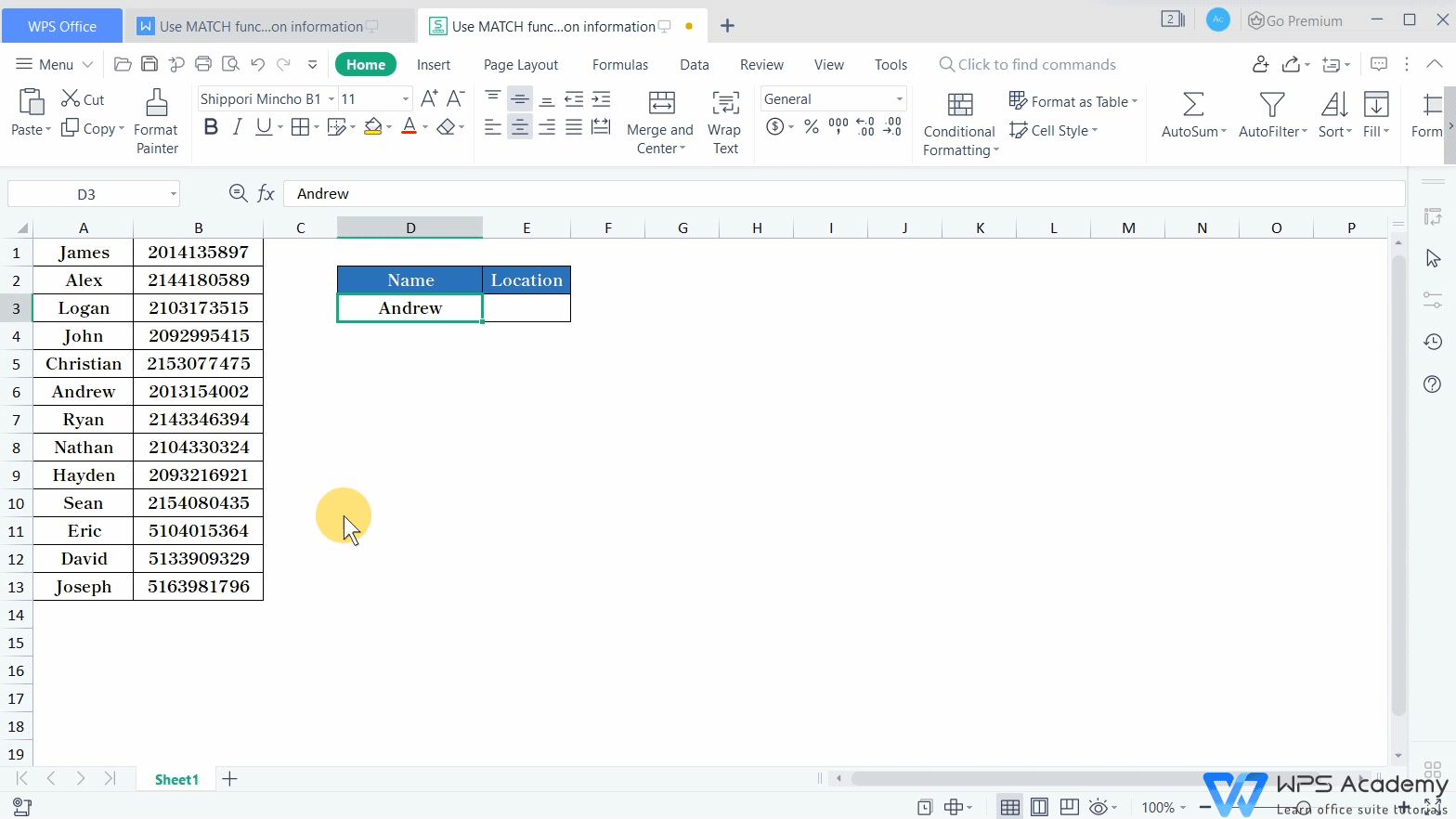In this table, we need to find out which row Andrew is located in column A by his name in cell D3. Click the Formulas tab and the Insert Function button to find the MATCH function.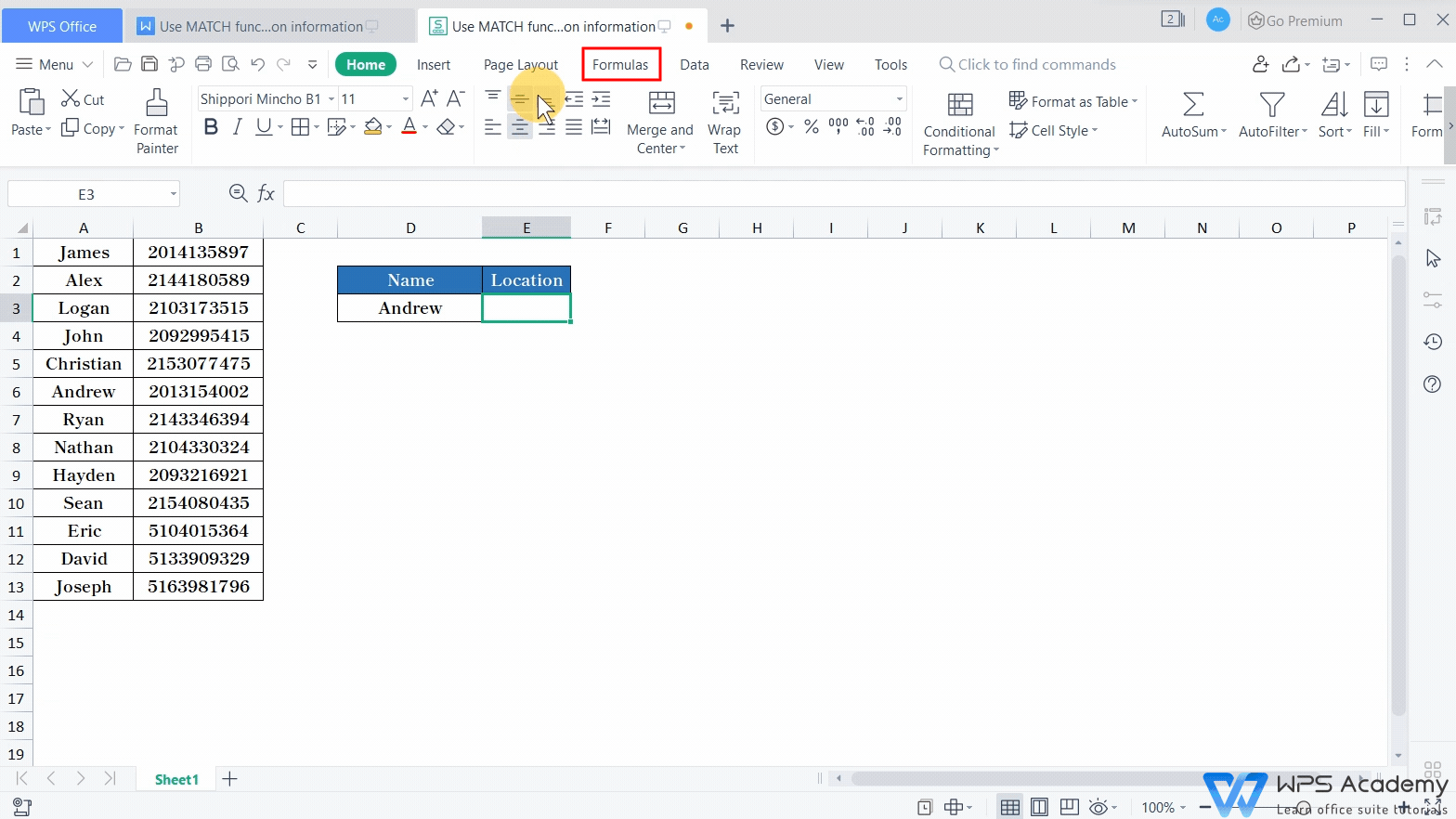The first parameter, Lookup_value is the specified lookup object, here our lookup object is Andrew, so we enter D3 at the first parameter.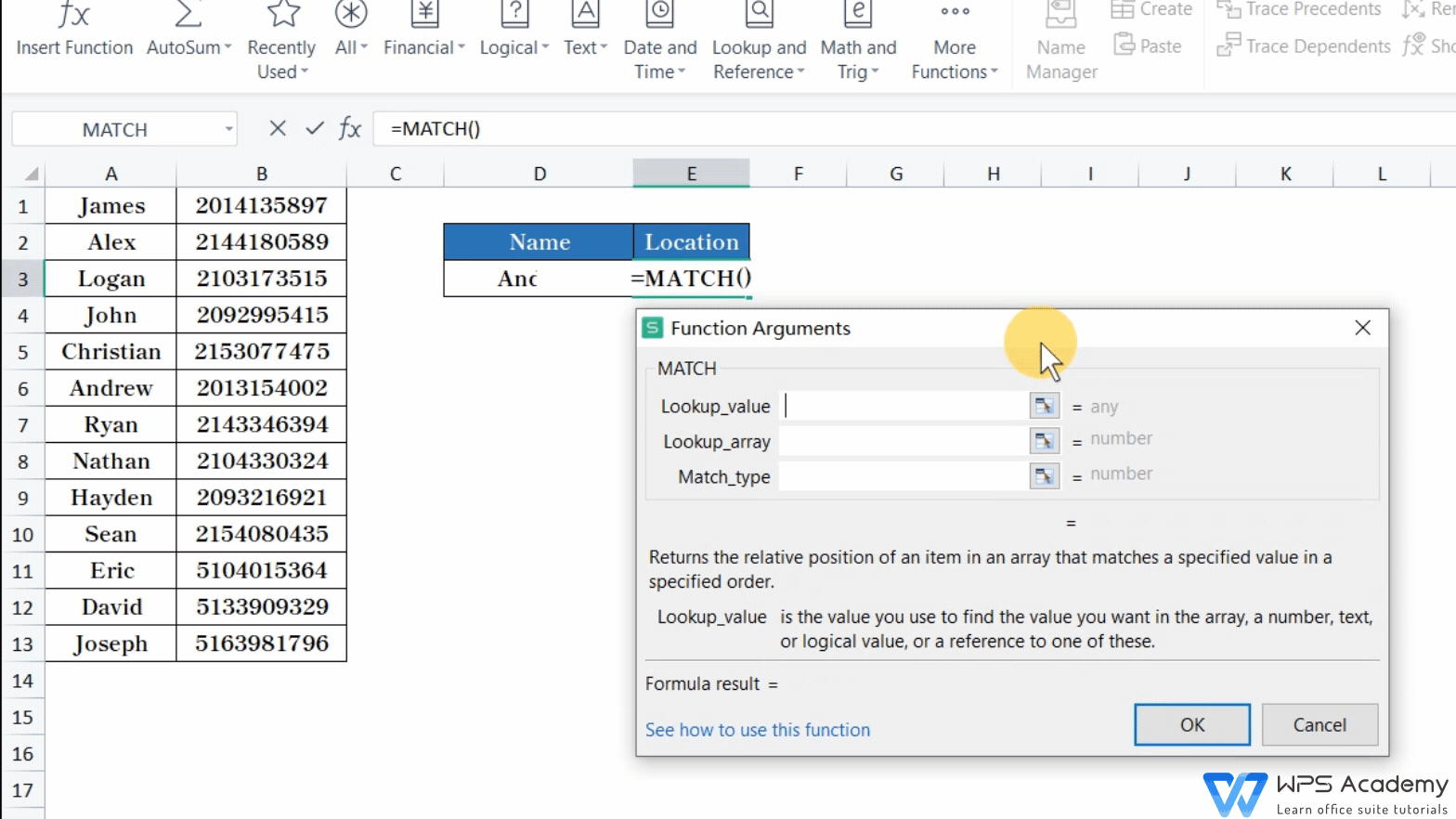The second parameter, Lookup_array is the cell region or array containing the object to find. It should be noted that the cell region or array can only be one column or one row. If there are multiple rows and columns, the MATCH function will return the error value #N/A. Here, the Lookup_array is column A, which contains names. So we select the name area A1:A13 in column A.The third parameter is Match_type, which is the matching pattern of lookup. The number 0 represents the exact match. 1 represents the largest value that is less than or equal to lookup_value, -1 represents the smallest value that is greater than or equal to lookup_value. We input 0 here. Then, click OK to complete the settings.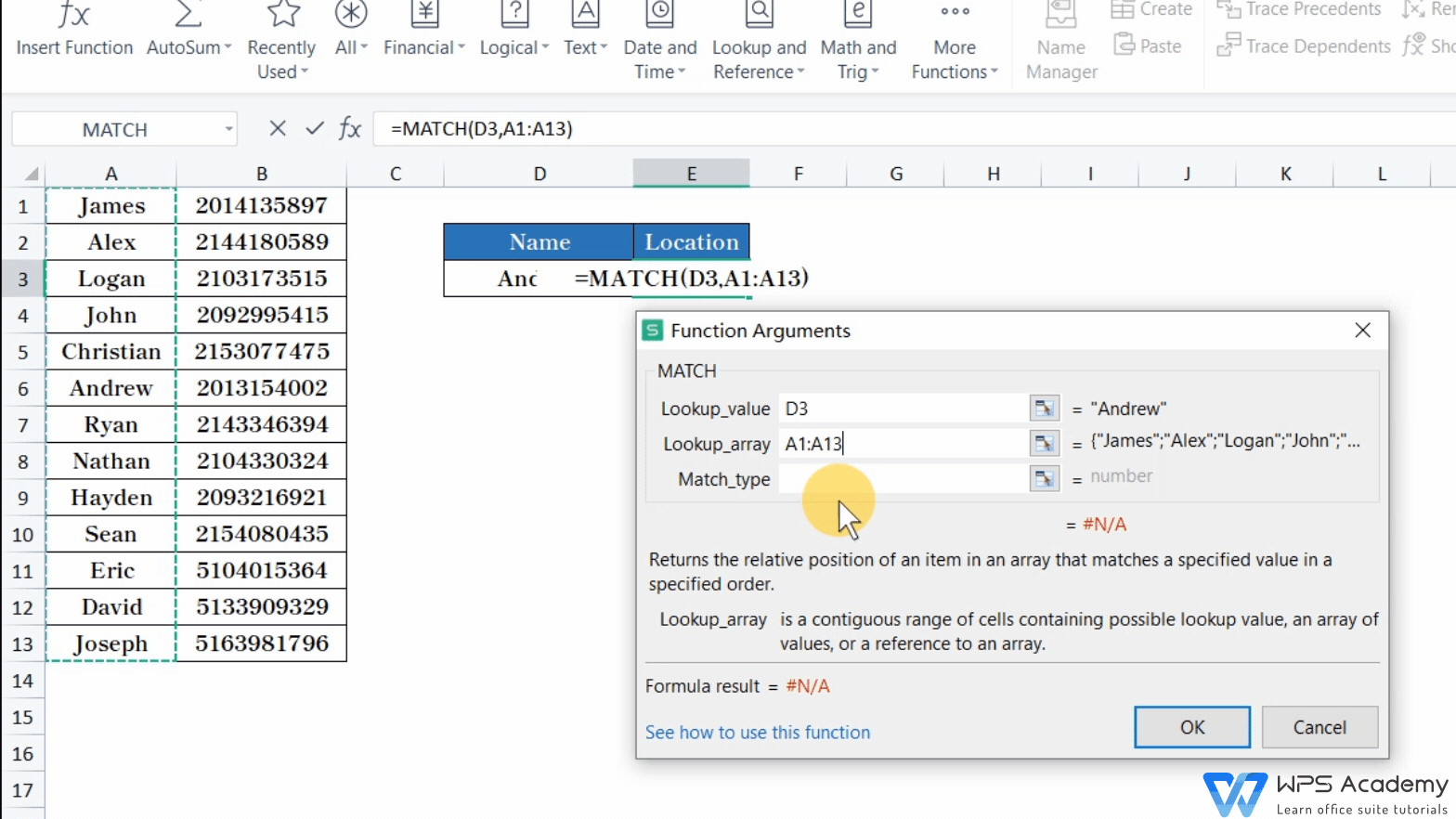The formula result is 6, which means that Andrew is in the sixth row of the A1:A13 cell range.

The return value 6 is the location information, which is related to the reference range of the second parameter. If we select A2:A13 at the second parameter, the return value is 5, which means the fifth row in the cell range A2:A13.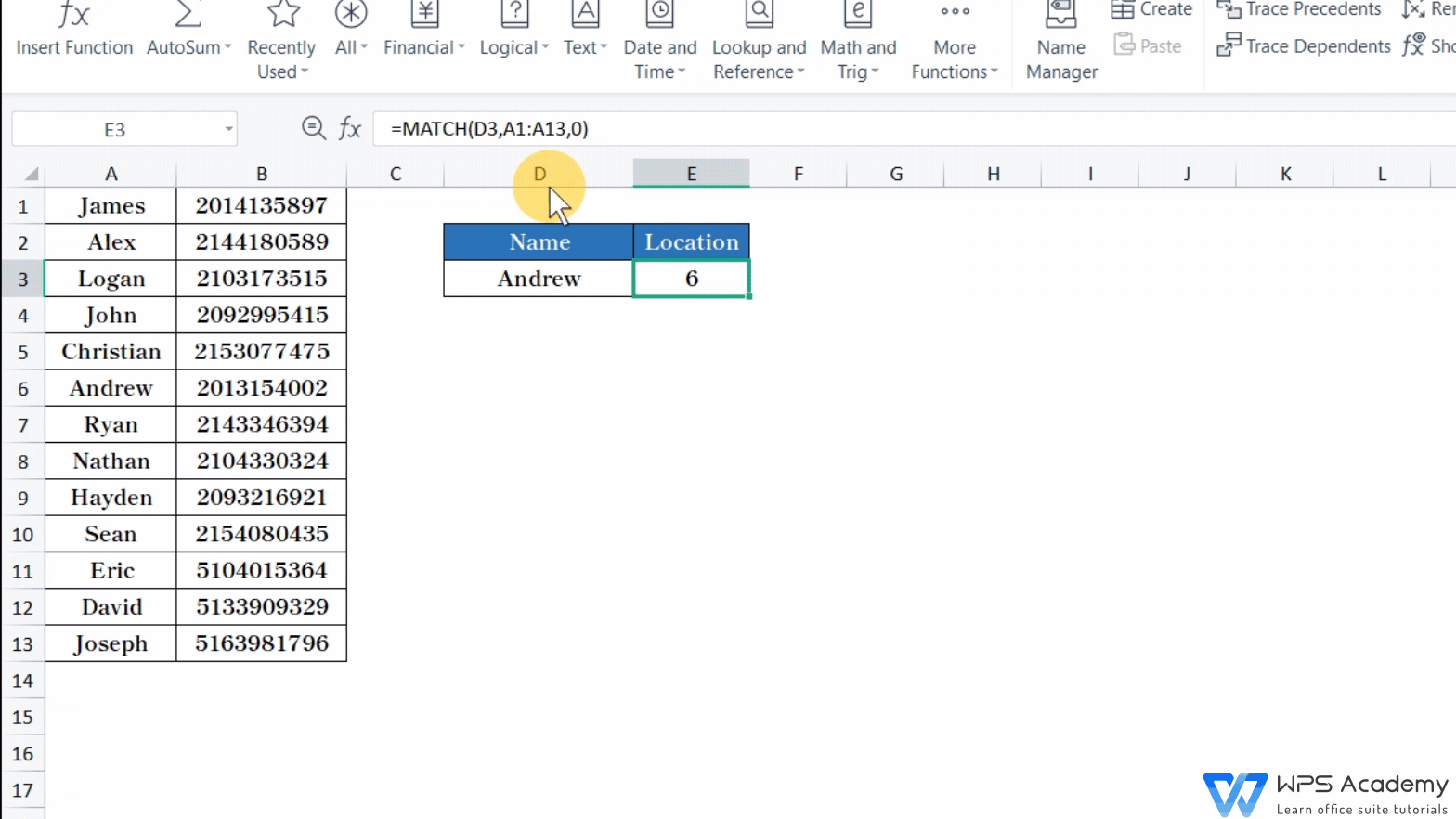Make your work easier with WPS Spreadsheet like Microsoft Excel. We can use the MATCH function and the INDEX function in combination, making it easier to look up the value.

For more details about the MATCH function, you're welcome to enter WPS Academy. Did you get it?